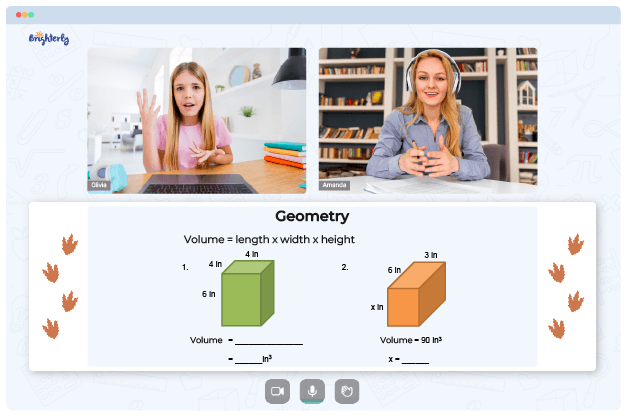# Types Of Angles Worksheets

Worksheets on angles are helpful to students who are learning about and practicing working with angles. Variations of this resource, like the classifying angles worksheet, contain tasks and questions where students should figure out and measure multiple angles. The next section will highlight some types of angles worksheets.

There are various angles worksheets, each with its characteristics and properties. Worksheets containing tasks on acute angles, right angles, obtuse angles, straight angles, and reflex angles are types of angles worksheet PDF.

A classify angles worksheet comes in handy for students learning different angles that an intersection of lines can create. Kids can learn about the properties of angles and how to classify them using examples and practice problems. Students using the naming angles worksheet will be shown an angle and asked whether it is acute, right, or obtuse.

Math for Kids

Is Your Child Struggling With Math?
1:1 Online Math TutoringGrade school students need to know about these angles because they’ll use the knowledge in many math and science topics. Practicing with worksheets will also improve their problem-solving abilities and let them understand the topic of angles deeply.

As students use angles worksheets, they learn about angles, their properties, and the ways to classify and measure them. These worksheets allow students to learn how to solve problems and apply what they’ve learned to real-world situations. If you want kids to be well-prepared for more advanced math concepts, consider getting them angles worksheets.### Types Of Angles Worksheets PDF

Types Of Angles Worksheet### Types Of Angles Worksheets PDF

Naming Angles Worksheet### Types Of Angles Worksheets PDF

Classifying Angles Worksheet### Types Of Angles Worksheets PDF

Types Of Angles Worksheet PDF

Need help with Geometry?• Does your child struggle with understanding of geometry?
• Try studying with an online tutor.

Is your child having difficulties mastering of geometry? An online tutor could provide the necessary guidance.